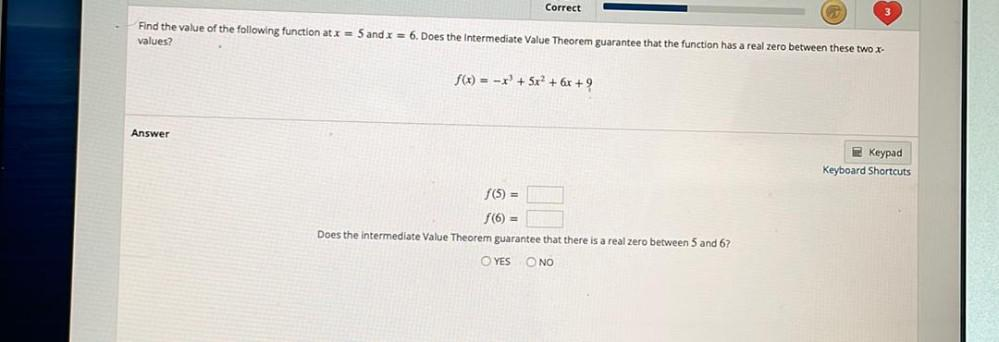Question:

# Correct Find the value of the following function at x = 5 and x = 6. Does the Intermediate Value Theorem guarantee that the funcCorrect Find the value of the following function at x = 5 and x = 6. Does the Intermediate Value Theorem guarantee that the function has a real zero between these two X- values? f(x) = -x + 5x2 + 6x + 9 Answer Keypad Keyboard Shortcuts f(5) = f(0) = Does the intermediate Value Theorem guarantee that there is a real zero between 5 and 6? YES NO